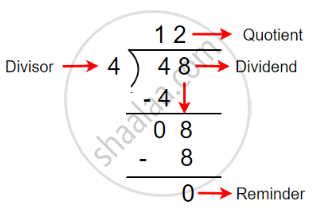# Concept of Divisor, Dividend

#### definition

Division: Division is an arithmetic operation that tells us the number of groups that can be made out of a number of factors or the number of multiples that should be there in a group.

Dividend: The word dividend means the number that is to be divided.

Divisor: In a division, the number that divides the dividend is called the divisor.

Divisible: A number is said to be divisible by another number, if, in the process of division, the remainder is zero.

Quotient: A quotient is a result obtained in the division process.

Remainder: Remainder is the number that is left over after dividing.

# Concept of Divisor, Dividend:

• Division is an arithmetic operation that tells us the number of groups that can be made out of a number of factors or the number of multiples that should be there in a group.

• The symbol denotes division - ÷• Dividend: The word dividend means the number that is to be divided.

Divisor: In a division, the number that divides the dividend is called the divisor.

Divisible: A number is said to be divisible by another number, if, in the process of division, the remainder is zero.

Quotient: A quotient is a result obtained in the division process.

Remainder: Remainder is the number that is left over after dividing.

Dividend = Divisor × Quotient + Remainder.

• When we divide 48 by 4, the remainder is zero. Therefore, 4 is a factor of 48, and 48 is a multiple of 4.
• Factors of 48: 1, 2, 3, 4, 6, 8, 12, 16, 24, 48.
If you would like to contribute notes or other learning material, please submit them using the button below.

### Shaalaa.com

Concept of division [00:04:39]
S
0%